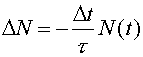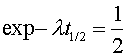Decay constants and half lives

Most nuclear decays occur independently (unlike those that occur in a chain reaction) where a given fraction of nuclei decay in a given time, independent of the number of nuclei. In a time Dt the number DN that decay are:The number that have not decayed at time t is N(t) and t is the exponential decay time. It is termed "exponential" because the solution to the above equation is:where N0 is the number of undecayed nuclei at t = 0. The symbol l = 1/t is known as the decay constant.

A half-life is the time it takes for half of the nuclei to disappear. At that point N(t) is one half of N0:Taking the logarithm of both sides of the above equation, gives the half life t1/2 in terms of the exponential time t.Working problems with exponential decays are good practice for many other fields. Exponential growth and decay are common concepts in biology, economics, and other sciences.